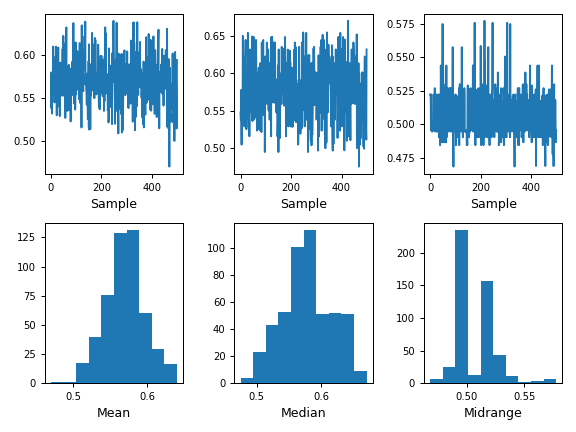### Search

Enter search terms or a module, class or function name.

# pandas.plotting.bootstrap_plot¶

pandas.plotting.bootstrap_plot(series, fig=None, size=50, samples=500, **kwds)[source]

Bootstrap plot on mean, median and mid-range statistics.

The bootstrap plot is used to estimate the uncertainty of a statistic by relaying on random sampling with replacement . This function will generate bootstrapping plots for mean, median and mid-range statistics for the given number of samples of the given size.

  “Bootstrapping (statistics)” in https://en.wikipedia.org/wiki/Bootstrapping_%28statistics%29
Parameters: series : pandas.Series Pandas Series from where to get the samplings for the bootstrapping. fig : matplotlib.figure.Figure, default None If given, it will use the fig reference for plotting instead of creating a new one with default parameters. size : int, default 50 Number of data points to consider during each sampling. It must be greater or equal than the length of the series. samples : int, default 500 Number of times the bootstrap procedure is performed. **kwds : Options to pass to matplotlib plotting method. matplotlib.figure.Figure Matplotlib figure.

See also

DataFrame.plot
Basic plotting for DataFrame objects.
Series.plot
Basic plotting for Series objects.

Examples

>>> s = pd.Series(np.random.uniform(size=100))
>>> fig = pd.plotting.bootstrap_plot(s)  # doctest: +SKIPScroll To Top